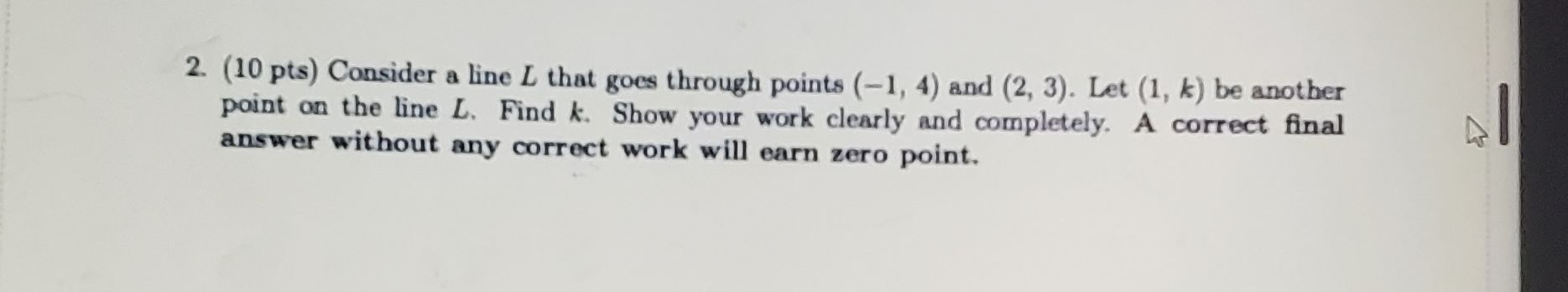### ¿Todavía tienes preguntas de matemáticas?

Pregunte a nuestros tutores expertos
Algebra
Pregunta2. $$( 10$$ pts $$)$$ Consider a line $$L$$ that goes through points $$( - 1,4 )$$ and $$( 2,3 )$$ . Let $$( 1 , k )$$ be another point on the line $$L$$ . Find $$k$$ . Show your work clearly and completely. A correct final answer without any correct work will earn zero point.

$$y = - \frac { 1 } { 3 } x + \frac { 11 } { 3 }$$
k=$$\frac{10}{3}$$